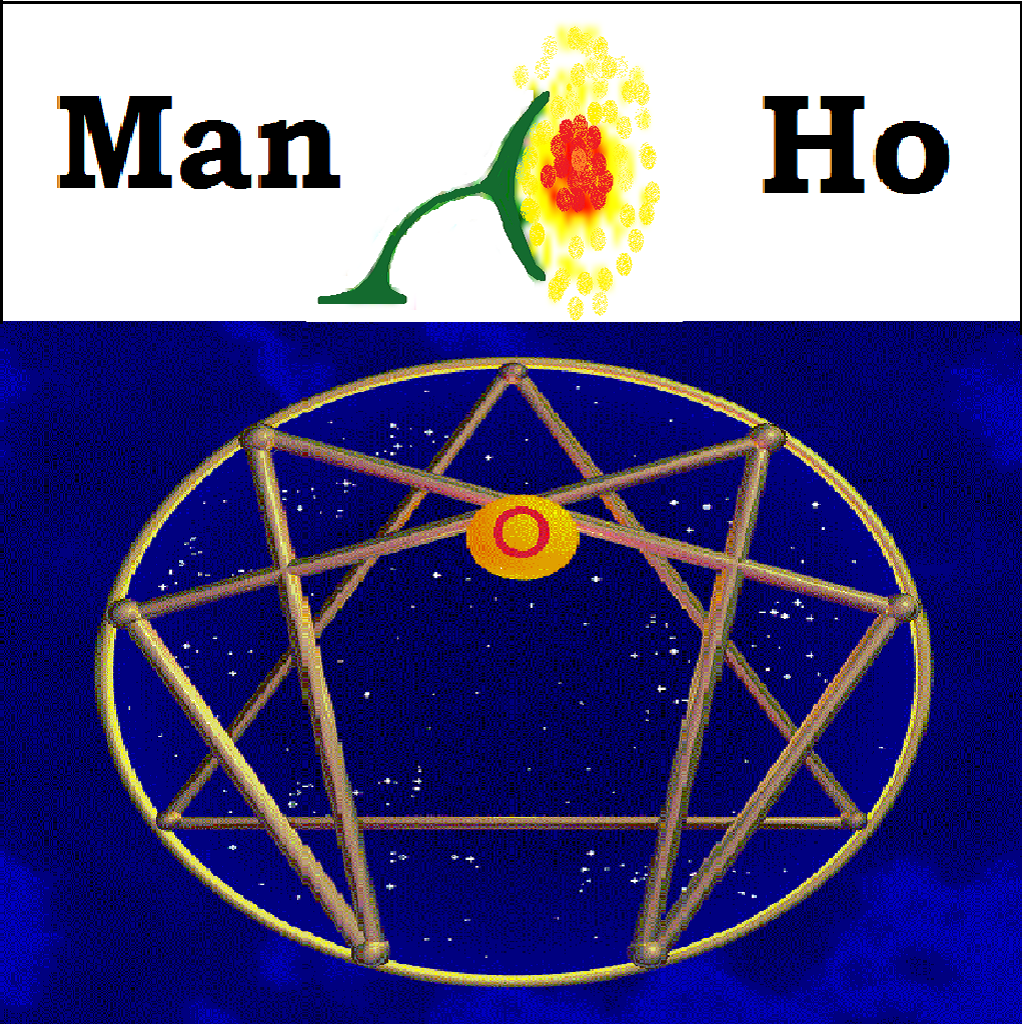THE FOURTHWAY MANHO E-JOURNAL
Volume 63       July 19, 2019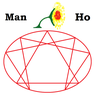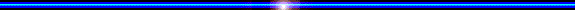MODERN MATHEMATICS AND THE STOPINDER NATURE OF THE SET OR CLASS THEORY

By Professor  Dr. Tan Man-Ho

(An excerpt from the original work, Real World Views, Book 2 by Professor  Dr. Tan Man-Ho entitled "Biocosmic Nervo-Reflectant and the Theory of Material Reflection in Man, Inner Development and Social Upheavals",  July  1972 ~ September 1973 Discourses, Chapter 7, Section C: "Modern Mathematics and the Stopinder Nature of the Set Theory", pp. 173~183)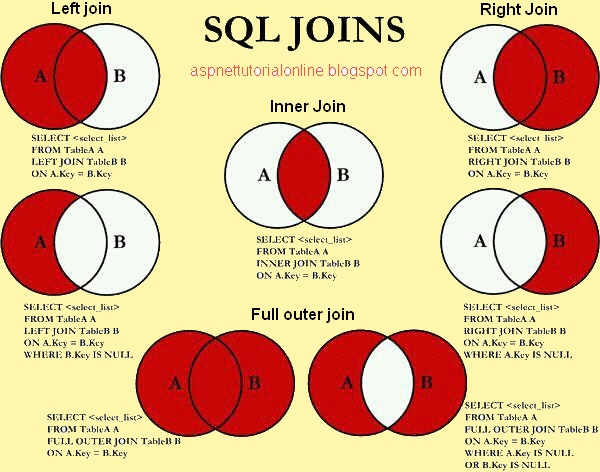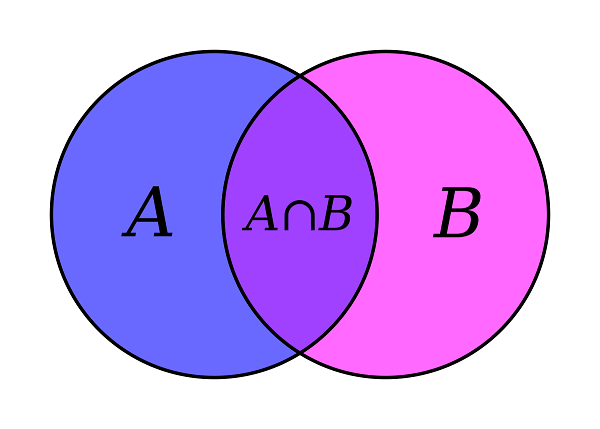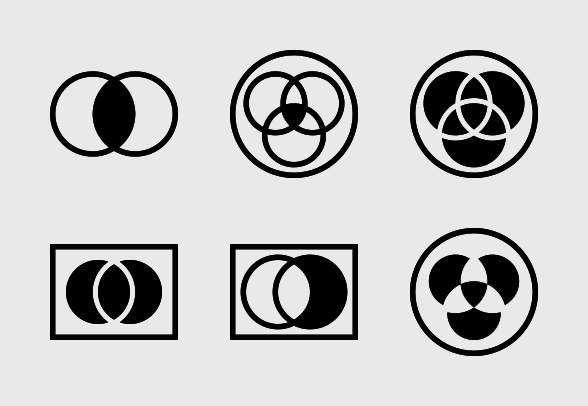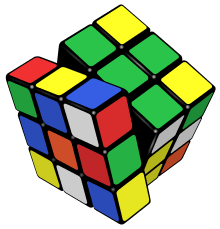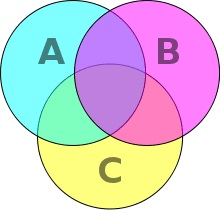C. MODERN MATHEMATICS AND THE STOPINDER NATURE OF THE SET OR CLASS THEORY

1 Back in the 1960's  the modern mathematics, properly so-called has ultimately proved itself to provide the exposition of the dialectic and mentation by forms – nature is looked upon as consisting of discrete items with distinct identity.  The Soviets during its Golden Age of Mathematics took the lead and the West badly needed to catch up – the sooner the better.  The general way which modern mathematics handles quantities (but not as yet fully develop quanta) takes a peculiar form, comparable to that used by a dialecticians in their listing and categorization of the entities in the real world.  A mathematical stopinder or class conception is observed in the new Set Theory, one of the many moderns of the Modern Mathematics from the Soviets who at that time were highly conscious of the class struggle processes of social development.

The conception of sets, resolutions of subsets into universal sets via limitation and delimitation through universality, that negative numbers and positive numbers are the same, that negative of +a is -a and negative of -a is +a, that subsets of a set are specific in numbers, that they are the qualities imported to a set i.e. { }, {a}, {b}, {c}, {a, b}, {b, c}, {c, a}, {a, b, c} are the subsets of the set {a, b, c}, whilst {a, b, c} as subsets is the nodal dialectical ‘leaping’ line of which {a, b, c, d}, that things(or numbers) have peculiar reflection and symmetry, etc. etc. are in fact all the earmarks of dialectic and the law of categorization.

A prime number is a whole number which is divisible only by itself and 1.

This is the definition, that every whole number divisible by itself and 1 must be a prime number.  Now to say that 1 which have all the characteristics of a prime number, to be a non-prime number is a self-contradiction, i.e. violates the earlier definition of a prime number.  It is assumed that 0 and 1 are something extra or super or non-prime number of which the reason for this assumption is unfounded.

According to this, therefore,

The set of positive prime numbers = {2, 3, 5, 7, 11 ……. }

The set of negative prime numbers = {-2, -3, -5, -7,-11 ……. }

And –1, 0, and 1 will be non-prime numbers.   But –1, 0, 1 are by no means, the neutral of the two sets of prime numbers, positive and negative.

The self-contradiction can be removed, if the particulars are put thus:

The set of positive prime numbers = {1, 2, 3, 5, 7, 11 ……. }

The set of negative prime numbers = {-1, -2, -3, -5, -7, -11 ……. }

‘0’ is not a prime number which is neutral to the two sets since ‘0’ can be divided by 2, 3, 4, etc.  (On the minor contradictions in modern mathematics, Jan 1975)

Sets cannot be anything else than a limitation of qualities which in its turn has within itself the tension to ‘push’ the limit into its infinite into universality, that line of limitation are ‘pushed’ to its terminal position of which something cannot keep it in the set anymore but must be distinguished from another.  The very end point of every set is its universality or infinity of elements or members, the only transformation into a oneness, which is the ultimate whole – the Universal Set is still not the Final Absolute Universal Set of the Real World.  But ordinary set theory is only the exposition of quanta into oneness, which must return to proceed to the next—to something without quanta—to quality.

The movement of set methodology [in the brain], inherent in set thinking, produce a ‘new’ form of classification of mathematics concern — the very form which penetrate in every qualitative distinction of quantities.  The further development of set theory is a resolution of itself into essence, into a substance essential to its existence, to the oneness of its practice, that of ‘Set Conception’— viz. detecting set, recognizing the ‘truth of imagination’ of absolute equality of set A and set B or ‘equal members’ although no mathematicians have ever i.e. intersection, manipulation of set lines or limitations to elements and called them union, etc. discover two things which are one and in fact the same thing.  C = C, it is said, but actually the first ‘C’ is never the same as the second ‘C’ and only that they look like the same one or else no distinction of the first C and the second C can ever be made.  Their equality implies that they have a common ‘something’ such as a value or number which makes them equal.  It is that they are 2 different identities wit he same algebraic representation.

The same goes to {a, b} = {a, b} where thorough ‘likeness’ is never the case.

2  The Twofold ‘Expression’ Of Intersection Of Sets

1.    {a,b,c} ∩ {c,d,c} = {c}

[Method of selecting the common element]

element same (D)              qualities different (f and w)

(i)   {Df,Df,Df,Df,Df,Df,Df} ∩ {Dw,Dw} = {Dfw ,Dfw}

Df is a Day of a week which is fine weather

Dw is a Day of a week which is windy weather

[The case when the elements are the same in both intersecting sets but of different qualities]

In Df,        f Є {D}   ⇔    D = {f}

In Dw,      w Є {D}  ⇔     D = {w}

In Dfw,      f Є {D}      and       w Є {D}    or     D = {f,w}

If D stand for a fine day, therefore f, and w are the two qualities of the element D.  Є is an element of the set of days (D)

(ii)   {Pmtc, Pmtc, Pmtc, Pmtc} ∩ {Pm,e,g, Pm,e,g} = {Pmtceg, Pmtceg}

if and only if the members of the second set are the concluding set, the member of the first set to form the standard set.

Criteria:

i. When intersection occurs between two sets of the same elements, same in the sense that they (element) are selected completely from a given standard set, the results gives a set of  the elements whereby each of which poses all the different qualities of the two intersecting parent set, and whose number is determined by the smaller set.

e.g  Standard Set: {B(f,h,b), B(f,h,b), B(f,h,b,s)}

Resolution into two sets and intersecting.

{B(f,h,b,s),B(f,h,b) ,B(f,h,b)} ∩ {B(f,h,b,s)} =  {B(f,h,b,s)}

B is the same element; f,h,b,s are qualities.  Note no repetition of f,h,b is allowed.  ∩ is the symbol for ‘intersection’.

ii.    {a,b,c}  ∩ {c,d,e} = {c}.

transposing,

{c} = {a,b,c} ∩ {c,d,e}

⇔    {c} = {c,a,b} ∩ {c,d,e}

⇔    {c} = {c, L}  ∩ {c, N},

where L and N are two sets not having common elements.

Also     {D(f,w)} = {D(f,w), Df} ∩ {D(f,w), Dw}

Transposing,

{D(f,w), Df} ∩ {D(f,w), Dw} = {D(f,w)}

D is called the standard set of reference.

e.g.       D = {3 days of a week}

= {Df, Dw, D(f,w)}

Where D(f,w), L is called the repetitive members of the standard sets, or members considered twice in the two process.  The repetitive members can have all even numbers for double intersection;

2.    Venn Diagram

(a)   {c} = {c,L} ∩ {c,N}       E = Standard Set of Reference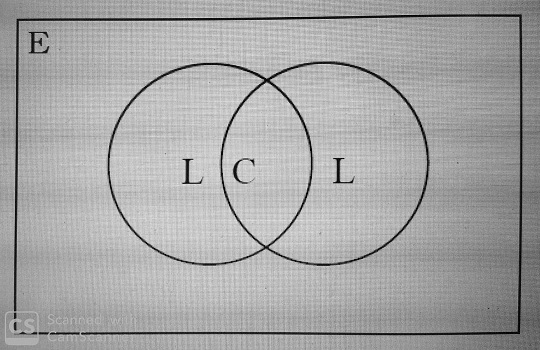(b)   {D(f,w)} = {D(f,w),Df} ∩ {D(f,w),Dw}

E = D = Standard Set of Reference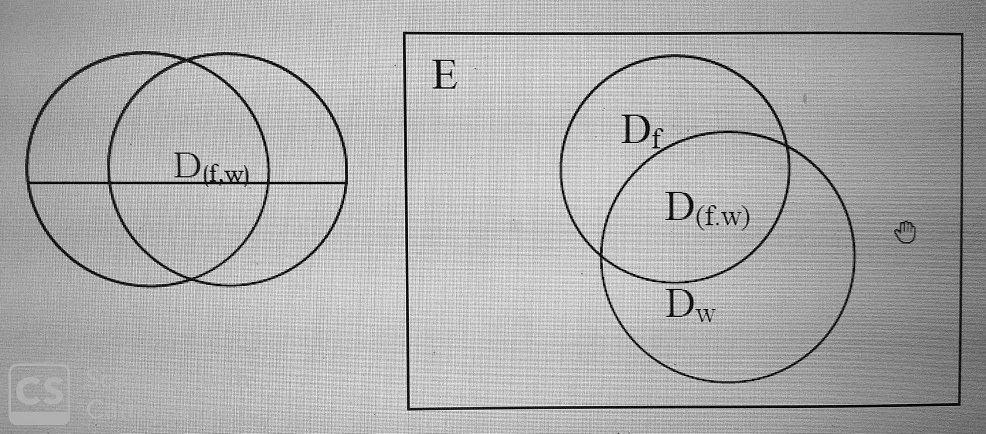e.g  of 25 girls, 18 like pop music,13 like classical music, and 10 like both.

i.e.

{10Gb} = {10Gb, 8Gp} ∩ {10Gb, 3Gc}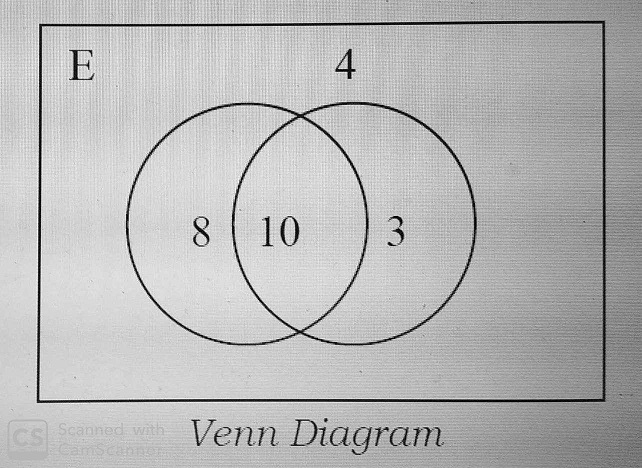3.    Procedure To Comprehension

[Building up the whole through the parts]

(A)   One is familiar that A ∩ B gives C and concretely,

{a,b,c} ∩ {c,d,e} = {c}.

that is, the intersection of A and B vanish into C….. now, given set C.

i.e        {c}.

{c} = {c,a,b} ∩ {c,d,e}

Now transform the subsets {a,b} and {d,e} into the abstract into L and M, we have,

{c} = {c,L} ∩ {c,M}

where, L and M are sets which do not have any same elements

Note: This backward ‘Faustian Movement’ has its utility where we deal with such problems:

For example:

There are 20 boys in a certain class. 16 of them study physics, 14 study chemistry, and 12 study both physics and chemistry.

In this case, {c} becomes

{12} = {12,4} ∩ {12,2}

where

A = {people taking both chemistry & physics, people taking physics}

B = {people taking both chemistry & physics, people taking chemistry}

C = {people taking both chemistry & physics}

(B) When this has been done the construction of Venn diagram is easier.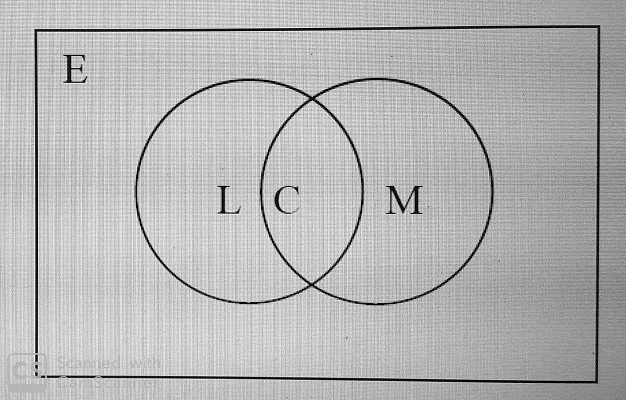E is the universal set to limit or give a name to the set in reference.

L,C,M is equal to the number of times different selections are made on E.

The Venn diagrams for {12} = {2,4} ∩ {12,2}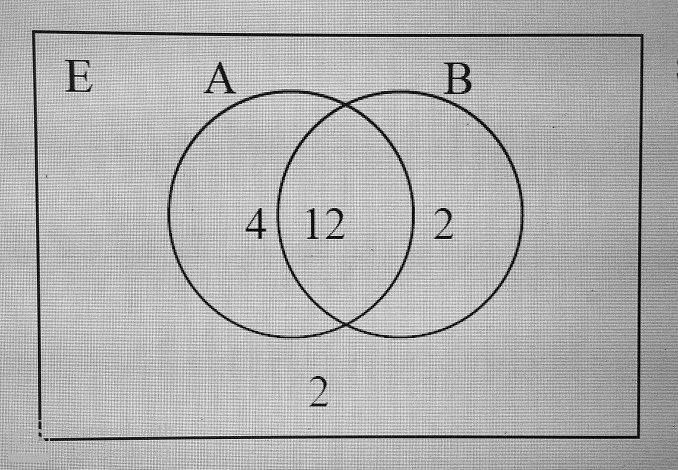Since, E = {20 pupils}

Remark:     G = {pupils wearing glasses} and W = {pupils wearing watches}. G ∩ W is not well defined because it is hardly known that whether there is a common element or not.

If on the other hand,

G = {pupils wearing glasses in the class, counted without respect to anything else not bothering about these with watches} and W = {pupils wearing watches in the same class, counted again without respect to anything else not bothering about those with glasses}, then G ∩ W has possible common element, if there is intersection, then realization occurs.

3   The relation between the old set theory and the new equivalent set theory, is as such that the old, much attributed to Boolean algebra of sets (George Boole) and Venn Diagram (John Venn), is algebraic, statistical and pictorial in its set to set relation whilst the new, due to application of Hegelian notion, etc, is dynamical and mentation by forms have found its way back into mathematics as a new complement of logical mathematical thoughts.  The dynamical set to set relations from the low sets, i.e. sets with limited number of elements in them, up to the temporally universalis and its final finite and infinity nature of both the case in the set theory and the real world as it is.  This is due to certain confusion about the universal set(s) — which often appears as the only universal set but as such is not and passes over into more universal sets and so on to infinity.  This limitation and delimitation is a movement of the logical conception of universality of set.  Discontinuities in the progression are subjected by the discontinuity intervals in the Octave of progression of set into its universality.

The fact that a set must be transformed into a subset before it becomes a subset of a more universal set is inevitable, because by doing so, the transformed-subset presupposes the existence of a qualitative from of which this subset is only a belonging-to or a “member” to another bigger qualitative subset which becomes the Universal set.  Whilst the transformation of this first Universal set into a fact, universal subset presupposes a universality of a higher qualitative form, the second universal set, etc., etc.

The inability to conceive motion, is apparently revealed when a teacher discusses the problem on the universal sets, the true significant of it is a formal logical habit of thinking has imposed itself on the dialectical and forms’ mentation in the head of the teacher.

A common misunderstanding about limitation and delimitation of universal set is expressed here.  A set, {a, b, c}, is a subset of the universal set {a, b, c…x, y, z}.  This limitation, or rather first limitation is itself a set {a, b, c…x, y, z} and its becoming of a subset of another universal set is unequivocally possible.  This set, can be the subset of another or rather the second universal set, {a, b, c...x, y, z ,of English alphabets, a, b, c…x, y, z of the Chinese Phonetic alphabet,…}, which consists of all known species of alphabets.  The initial limitation becomes delimitation and propels into more logical universal sets – universality with boundaries to fence more dialectical “notes” and mathematical “stopinders”, which still preserves the weak oneness.  (Dialectic, Stopinders and Sets)

 Home Matrix Chart Founder Activities Seminars Publication MRD MMC MTD MHE HEIGRENS World Peace Odyssey Holistic Point E-Journal Stopinder Announcement FM Studio Links Contact